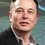# Prove it

Prove that

$$\tan n\theta =\large{ \frac{ \binom{n}{1}t - \binom{n}{3}t^{3} + \binom{n}{5}t^{5} - ...........}{ 1 - \binom{n}{2}t^{2} + \binom{n}{4}t^{4} - .........................}}$$

where $t = \tan \theta$Note by U Z
6 years, 8 months ago

This discussion board is a place to discuss our Daily Challenges and the math and science related to those challenges. Explanations are more than just a solution — they should explain the steps and thinking strategies that you used to obtain the solution. Comments should further the discussion of math and science.

When posting on Brilliant:

• Use the emojis to react to an explanation, whether you're congratulating a job well done , or just really confused .
• Ask specific questions about the challenge or the steps in somebody's explanation. Well-posed questions can add a lot to the discussion, but posting "I don't understand!" doesn't help anyone.
• Try to contribute something new to the discussion, whether it is an extension, generalization or other idea related to the challenge.
• Stay on topic — we're all here to learn more about math and science, not to hear about your favorite get-rich-quick scheme or current world events.

MarkdownAppears as
*italics* or _italics_ italics
**bold** or __bold__ bold
- bulleted- list
• bulleted
• list
1. numbered2. list
1. numbered
2. list
Note: you must add a full line of space before and after lists for them to show up correctly
paragraph 1paragraph 2

paragraph 1

paragraph 2

[example link](https://brilliant.org)example link
> This is a quote
This is a quote
    # I indented these lines
# 4 spaces, and now they show
# up as a code block.

print "hello world"
# I indented these lines
# 4 spaces, and now they show
# up as a code block.

print "hello world"
MathAppears as
Remember to wrap math in $$ ... $$ or $ ... $ to ensure proper formatting.
2 \times 3 $2 \times 3$
2^{34} $2^{34}$
a_{i-1} $a_{i-1}$
\frac{2}{3} $\frac{2}{3}$
\sqrt{2} $\sqrt{2}$
\sum_{i=1}^3 $\sum_{i=1}^3$
\sin \theta $\sin \theta$
\boxed{123} $\boxed{123}$

## Comments

Sort by:

Top Newest

By De Moivre's theorem

$(cos\theta + i sin\theta)^{n} = cos(n\theta) + i sin(n\theta)$

Writting the binomial expression of

$(cos\theta + i sin\theta)^{n}$

Now

Equating real part to $cos(n\theta)$

And imaginary part to $sin(n\theta)$

We get(let cos = c, sin = s)

$\displaystyle c(n\theta) = c^{n} - C_2^{n} c^{n -2} s^{2} +\ldots$

$\displaystyle s(n\theta) = C_1^{n} c^{n-1} s - C_3^{n}c^{n-3} s^{3} + \ldots$

Dividing equation 2 by 1 we get

$\displaystyle t(n\theta) = \frac{C_1^{n} c^{n-1}s - C_3^{n} c^{n-3}s^{3} + \ldots}{c^{n} - C_2^{n}c^{n-2}s^{2} + \ldots}$

Now divide by $\cos^{n} \theta$ in numerator and denominator to get the required expression.

Hence Proved!

- 6 years, 8 months ago

Log in to reply

Thanks Good solution

- 6 years, 8 months ago

Log in to reply

Please help

- 6 years, 8 months ago

Log in to reply

you too please help

- 6 years, 8 months ago

Log in to reply

@Sanjeet Raria You too please

- 6 years, 8 months ago

Log in to reply

@U Z @Mursalin Habib @John Muradeli @Cody Johnson You too please help

To Brilliant.org ,

Here after a comment is posted and when we click the edit button and want mention someone the menu is not coming . we have to make a new comment for this

For example firstly I forget about the people in the second, third and four comment and when i clicked the edit and type their name the menu was not displayed

- 6 years, 8 months ago

Log in to reply

@U Z Yes that's an issue - what you need to do, as far as I'm as a non-moderator am concerned, is mention all the names first, in the order you want, and only then type your text. If text needs to be between the names, space the names appropriately.

This does need to be fixed, though. I thought I was the only one, but apparently not.

- 6 years, 8 months ago

Log in to reply

I would solve this one by using De Moiver's identity (cosθ+jsinθ)^n=cosnθ+jsinnθ. Then tannθ=sinnθ/cosnθ. By expanding into powers we have (cosθ+jsinθ)^n=Sum(j^k *sin(θ)^k * cos(θ)^(n-k) * (n per k) )=Sum(j^k *tan(θ)^k * cos(θ)^n * (n per k) ). By grouping into real and imaginary parts we can get the result given. (I should learn to write in these posts in a more beautiful way :))) )

- 6 years, 6 months ago

Log in to reply

thanks for proving, refer this

For posting problems with mathematical expressions refer thisFor a complete guide for higher mathematical expressions refer This Wikibook

An example -Writing this we get,You can refer others too ,

Right Click a problem and select open in a new windowNow just copy it your job becomes easierFor posting images in a comment write

! [Description or leave it blank] (Url of the image) ( don't leave any gap here)

For posting question and solutions its not needed as you now @Nicholas Nye

- 6 years, 6 months ago

Log in to reply

Thanks!!

- 6 years, 6 months ago

Log in to reply

tan (A+B+C+......) = $\frac{S_{1}-S_{3}+S_{5}-.....}{1-S_{2}+S_{4}-......}$

Where $S_{i}$ is sum of products of i terms taken at a time.

For A=B=C...=$\theta$, You will get desired result!

- 6 years, 8 months ago

Log in to reply

×

Problem Loading...

Note Loading...

Set Loading...